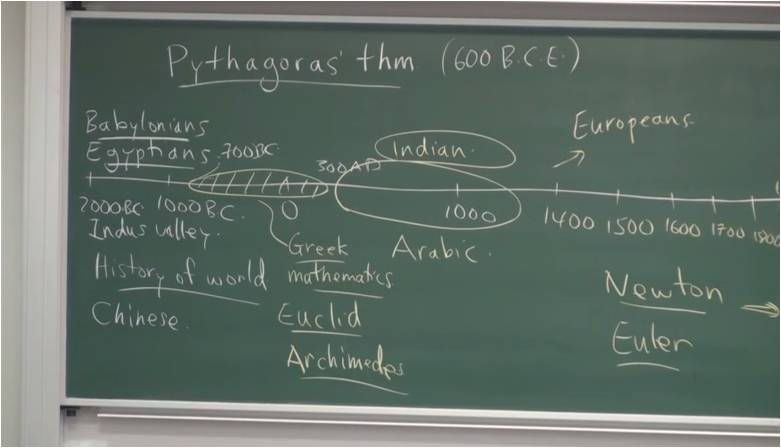# InfoCoBuild

## MATH 3560 - History of Mathematics

MATH 3560: History of Mathematics (UNSW). Taught by Professor N. J. Wildberger, this course provides an overview of the history of mathematics, in 17 lectures; meant for a broad audience, not necessarily mathematics majors. Starting with Greek mathematics, Professor N. J. Wildberger discusses Hindu, Chinese and Arabic influences on algebra; the development of coordinate geometry, calculus and mechanics; the course of geometry from projective to non-Euclidean in the 19th century; complex numbers and algebra; differential geometry; and topology. This course roughly follows John Stillwell's book 'Mathematics and its History' (Springer, 3rd ed).Lecture 1a - Pythagoras' Theorem Lecture 1b - Pythagoras' Theorem (cont.) Lecture 2a - Greek Geometry Lecture 2b - Greek Geometry (cont.) Lecture 3a - Greek Number Theory Lecture 3b - Greek Number Theory (cont.) Lecture 04 - Infinity in Greek Mathematics Lecture 5a - Number Theory and Algebra in Asia Lecture 5b - Number Theory and Algebra in Asia (cont.) Lecture 6a - Polynomial Equations Lecture 6b - Polynomial Equations (cont.) Lecture 7a - Analytic Geometry and the Continuum Lecture 7b - Analytic Geometry and the Continuum (cont.) Lecture 08 - Projective Geometry Lecture 09 - Calculus Lecture 10 - Infinite Series Lecture 11 - Mechanics and the Solar System Lecture 12 - Non-Euclidean Geometry Lecture 13 - The Number Theory Revival Lecture 14 - Mechanics and Curves Lecture 15 - Complex Numbers and Algebra Lecture 16 - Differential Geometry Lecture 17 - Topology